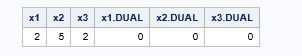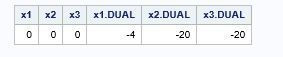## purpose of FIX command in proc optmodel for solving non linear optimization problem

hi,

I am trying to solve the following problemI mean I want to minimize the function f(x).

I have written the following code (without the FIX commands)

```proc optmodel;
var x1, x2 , x3;
min f = x1^2 + 2*(x2^2) + 5*(x3^2) -4*x1 -20*x2 -20*x3;
/* starting point */
x1 = 0;
x2 = 0;
x3 = 0;
solve;
print x1 x2 x3 x1.dual x2.dual x3.dual;
quit;```

and I get the following resultThere are no values for the dual but I get the value of objective function

But when I write the same code with fix commands (as given below).

``````proc optmodel;
var x1, x2 , x3;
min f = x1^2 + 2*(x2^2) + 5*(x3^2) -4*x1 -20*x2 -20*x3;
/* starting point */
fix x1 = 0;
fix x2 = 0;
fix x3 = 0;
solve;
print x1 x2 x3 x1.dual x2.dual x3.dual;
quit;``````

then i get the following result+

now there are no values for the 3 variables and also no value for the objective function

Sorry I dont know exactly the function of "FIX" command.

Please can you explain the purpose of "FIX" command here.

Which code should I use to solve this problem?

thanks

1 ACCEPTED SOLUTION

Accepted Solutions

## Re: purpose of FIX command in proc optmodel for solving non linear optimization problem

The FIX statement fixes variables to the specified values.  If you want to minimize the function, don't fix anything, and the solver will find optimal values for the variables.  At a locally optimal solution, the dual values will be 0.  But to find the gradient at a given solution, you must fix the variables.  The resulting dual values (gradient) indicate the direction of steepest descent.  As you did in other questions, you would proceed with a line search to find the best step length alpha in that direction.